# C# Multithreading Programming Tutorial with Examples

#### View more Tutorials: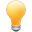Follow us on our fanpages to receive notifications every time there are new articles.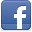Facebook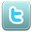Twitter

## 1- The concept of multithreading

Multithreading is an important concept in programming languages, and C# too, which is how to make the thread of the program running parallelly  to each other. For simplicity you see an example below:
``````
using System;
using System.Collections.Generic;
using System.Linq;
using System.Text;

{
{
public static void Main(string[] args)
{
// Creates a child thread, which runs in parallel with the main thread.

// In the main thread print out character '-'
for (int i = 0; i < 50; i++)
{
Console.Write('-');
// Sleep 70 millisenconds.
}
}
public static void WriteB()
{
// Print out the 'B' 100 times.
for (int i = 0; i < 100; i++)
{
Console.Write('B');

// Sleep 100 millisenconds
}
}
}
}
``````
And you run this class: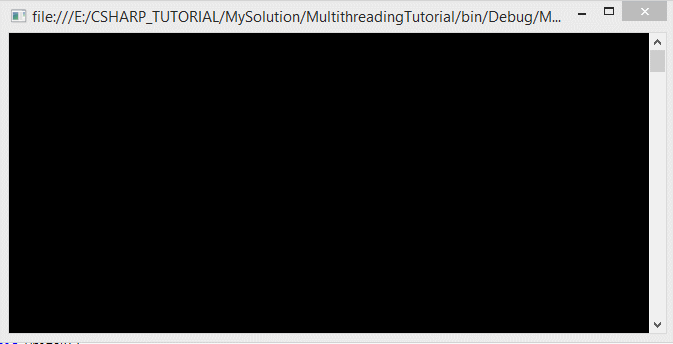The operating principle of the thread is explained in the following illustration: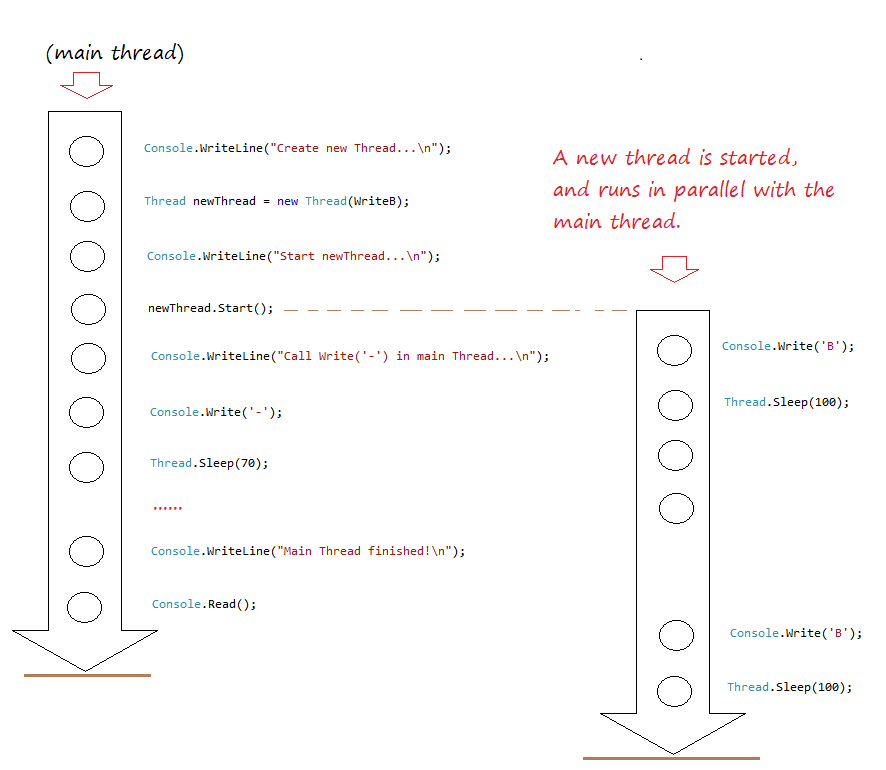## 2- Pass parameters to Thread

Above you have become familiar with HelloThread example, you have created an object wrapped  a static method to execute this method in parallel with the main thread.
Static method can become a parameter passed into  constructor of the Thread class if that method don't have parameters, or with a single parameter object type.
``````
// A static method, have no parameter.
public static void LetGo()
{
// ...
}

// A static method, has only one parameter type of object.
public static void GetGo(object value)
{
// ...
}
``````
This next example, I'll create a thread to wrap  a static method with one parameter (object type). Running thread and pass value to the parameter.
MyWork.cs
``````
using System;
using System.Collections.Generic;
using System.Linq;
using System.Text;

{
class MyWork
{
public static void DoWork(object ch)
{
for (int i = 0; i < 100; i++)
{
Console.Write(ch);
// Sleep 50 milliseconds
}
}
}
}
``````
``````
using System;
using System.Collections.Generic;
using System.Linq;
using System.Text;

{
{
public static void Main(string[] args)
{

// Create a Thread object to wrap around the static method MyWork.DoWork

// and pass parameter to MyWork.DoWork.

for (int i = 0; i < 20; i++)
{
Console.Write(".");
// Sleep 30 milliseconds.
}
}
}
}
``````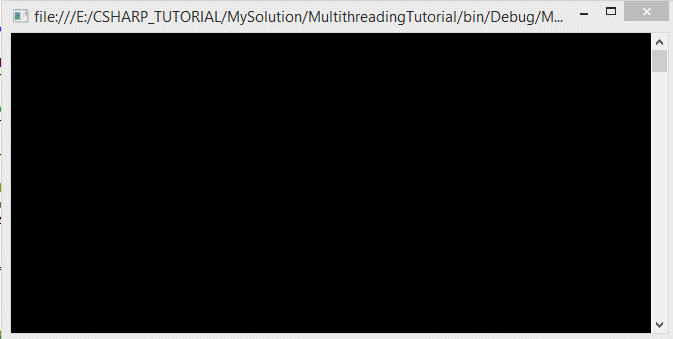## 3- Thread uses non-static method

You can also create a thread to execute (using) a none-static method. See for example:
Worker.cs
``````
using System;
using System.Collections.Generic;
using System.Linq;
using System.Text;
{
class Worker
{
private string name;
private int loop;
public Worker(string name, int loop)
{
this.name = name;
this.loop = loop;
}
public void DoWork(object value)
{
for (int i = 0; i < loop; i++)
{
Console.WriteLine(name + " working " + value);
}
}
}
}
``````
WorkerTest.cs
``````
using System;
using System.Collections.Generic;
using System.Linq;
using System.Text;

{
class WorkerTest
{
public static void Main(string[] args)
{
Worker w1 = new Worker("Tom",10);

// Pass parameter to DoWork method.

Worker w2 = new Worker("Jerry",15);

// Pass parameter to DoWork method.
}
}
}
``````
Running the example:
``````
Tom working A
Jerry working B
Jerry working B
Tom working A
Tom working A
Jerry working B
Tom working A
Jerry working B
Tom working A
Jerry working B
Tom working A
Jerry working B
Tom working A
Jerry working B
Jerry working B
Tom working A
Tom working A
Jerry working B
Jerry working B
Tom working A
Jerry working B
Jerry working B
Jerry working B
Jerry working B
Jerry working B
``````

ThreadStart is a Delegate, it is initiated by wrap a method. And it is passed as a parameter to initialize the Thread object.

With .Net < 2.0, to start a thread, you need to create ThreadStart, which is a delegate.

Beginning in version 2.0 of the .NET Framework, it is not necessary to create a delegate explicitly. You only need to specify the name of the method in the Thread constructor.
Programmer.cs
``````
using System;
using System.Collections.Generic;
using System.Linq;
using System.Text;

{
class Programmer
{
private string name;
public Programmer(string name)
{
this.name= name;
}
// This is none static method, no parameters.
public void DoCode()
{
for (int i = 0; i < 5; i++)
{
Console.WriteLine(name + " coding ... ");
}
}
}
}
``````
``````
using System;
using System.Collections.Generic;
using System.Linq;
using System.Text;

{
{
public static void Main(string[] args)
{
// Create a ThreadStart object that wrap a static method.
// (It can only wrap method have no parameters)

// Create Programmer object.
Programmer tran = new Programmer("Tran");

// You can also create a ThreadStart object that wrap a non-static method.
// (It can only wrap method have no parameters)

}
public static void DoWork()
{
for (int i = 0; i < 10; i++)
{
Console.Write("*");
}
}
}
}
``````
Running the example:
``````
*Tran coding ...
Tran coding ...
Tran coding ...
*Tran coding ...
*Tran coding ...
*******
``````

## 5- Thread with anonymous code

In the above, you have created  Thread using a specific method. You can create a thread to execute any code
``````
// Use delegate() to create anonymous method.
delegate()
{
//  ...
}
``````
Example:
``````
using System;
using System.Collections.Generic;
using System.Linq;
using System.Text;

{
{
public static void Main(string[] args)
{
// Creates a thread to execute a snippet code.
delegate()
{
for (int i = 0; i < 10; i++)
{
Console.WriteLine("Code in delegate() " + i);
}
}
);

// Creates a thread to execute a snippet code.
delegate(object value)
{
for (int i = 0; i < 10; i++)
{
Console.WriteLine("Code in delegate(object) " + i + " - " + value);
}
}
);

// Pass parameter to delegate().
}
}
}
``````
Running the example:
``````
Code in delegate() 0
Code in delegate(object) 0 - !!!
Code in delegate() 1
Code in delegate() 2
Code in delegate(object) 1 - !!!
Code in delegate() 3
Code in delegate(object) 2 - !!!
Code in delegate() 4
Code in delegate() 5
Code in delegate(object) 3 - !!!
Code in delegate() 6
Code in delegate() 7
Code in delegate(object) 4 - !!!
Code in delegate() 8
Code in delegate() 9
Code in delegate(object) 5 - !!!
Code in delegate(object) 6 - !!!
Code in delegate(object) 7 - !!!
Code in delegate(object) 8 - !!!
Code in delegate(object) 9 - !!!
``````

In multi-threaded programming, you can name the stream, it is really useful in case of debugging , to know the code that is being executed in which  thread.

See illustration:
``````
using System;
using System.Collections.Generic;
using System.Linq;
using System.Text;

{
{
public static void Main(string[] args)
{
// Set name to current thread

for (int i = 0; i < 5; i++)
{
}
}
public static void LetGo()
{
for (int i = 0; i < 10; i++)
{
}
}
}
}
``````
Running the example:
``````
Code of Main
Code of Main
Code of Let's Go
Code of Main
Code of Let's Go
Code of Main
Code of Main
Code of Main
Code of Let's Go
Code of Let's Go
Code of Let's Go
Code of Let's Go
Code of Let's Go
Code of Let's Go
Code of Let's Go
Code of Let's Go
``````

there are 5 priority of a thread in C # , they are defined in the ThreadPriority enum .
``````
Lowest,
BelowNormal,
Normal,
AboveNormal,
Highest
}
``````
Usually with high-speed computers, if the thread only execute little amount of work, you find it very difficult to detect the difference between high-priority threads and threads have low priority.

The example below has two threads, each prints the text of 100K lines (numbers large enough to see the difference).
``````
using System;
using System.Collections.Generic;
using System.Linq;
using System.Text;

{
{
private static DateTime endsDateTime1;
private static DateTime endsDateTime2;
public static void Main(string[] args)
{
endsDateTime1 = DateTime.Now;
endsDateTime2 = DateTime.Now;

// Set highest priority for thread1

// Set the lowest priority for thread2.
}
public static void Hello1()
{
for (int i = 0; i < 100000; i++)
{
Console.WriteLine("Hello from thread 1: "+ i);
}
// The time of thread1 ends.
endsDateTime1 = DateTime.Now;
PrintInterval();
}
public static void Hello2()
{
for (int i = 0; i < 100000; i++)
{
Console.WriteLine("Hello from thread 2: "+ i);
}
// The time of thread2 ends.
endsDateTime2 = DateTime.Now;
PrintInterval();
}
private static void PrintInterval()
{
// Interval (Milliseconds)
TimeSpan interval = endsDateTime2 - endsDateTime1;
}
}
}
``````
Running the example: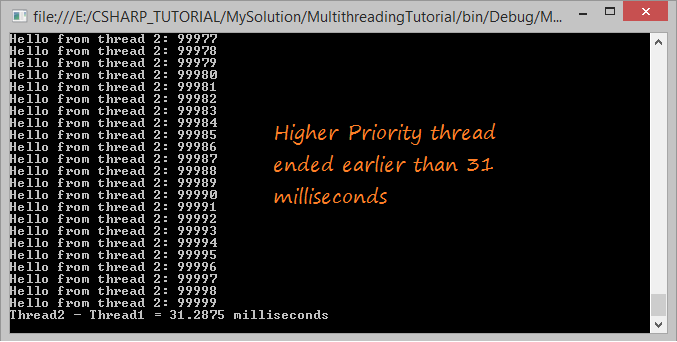## 8- Using Join()

``````
using System;
using System.Collections.Generic;
using System.Linq;
using System.Text;

{
{
public static void Main(string[] args)
{

// wait for the letgoThread to finish, then continue running.

// This statement must wait for letgoThread to end, then continue running.
}
public static void LetGo()
{
for (int i = 0; i < 15; i++)
{
Console.WriteLine("Let's Go " + i);
}
}
}
}
``````
Running the example:
``````
Let's Go 0
Let's Go 1
Let's Go 2
Let's Go 3
Let's Go 4
Let's Go 5
Let's Go 6
Let's Go 7
Let's Go 8
Let's Go 9
Let's Go 10
Let's Go 11
Let's Go 12
Let's Go 13
Let's Go 14
``````

## 9- Using Yield()

Theoretically, to ‘yield’ means to let go, to give up, to surrender. A yielding thread tells the operator system that it’s willing to let other threads be scheduled in its place. This indicates that it’s not doing something too critical. Note that it’s only a hint, though, and not guaranteed to have any effect at all.

so, Yield() methods is used when you see that thread is free, it's not doing anything important, it suggests operating system give priority temporarily to the other thread.
The example below, there are two threads, each thread print out a text 100K times (the numbers are large enough to see the difference). One thread is the highest priority, and other thread is lowest  priority. See completion time of 2 threads.
``````
using System;
using System.Collections.Generic;
using System.Linq;
using System.Text;

{
{

private static DateTime importantEndTime;
private static DateTime unImportantEndTime;

public static void Main(string[] args)
{
importantEndTime = DateTime.Now;
unImportantEndTime = DateTime.Now;

// Set the highest priority for this thread.

// Set the lowest priority for this thread.

}

// A important job which requires high priority.
public static void ImportantWork()
{
for (int i = 0; i < 100000; i++)
{
Console.WriteLine("\n Important work " + i);

// Notifying the operating system,
}
// The end time of this thread.
importantEndTime = DateTime.Now;
PrintTime();
}

public static void UnImportantWork()
{
for (int i = 0; i < 100000; i++)
{
Console.WriteLine("\n  -- UnImportant work " + i);
}
// The end time of this thread.
unImportantEndTime = DateTime.Now;
PrintTime();
}

private static void PrintTime()
{
// Interval (Milliseconds)
TimeSpan interval = unImportantEndTime - importantEndTime;

}

}

}
``````
Running class in the absence of Thread.Yield():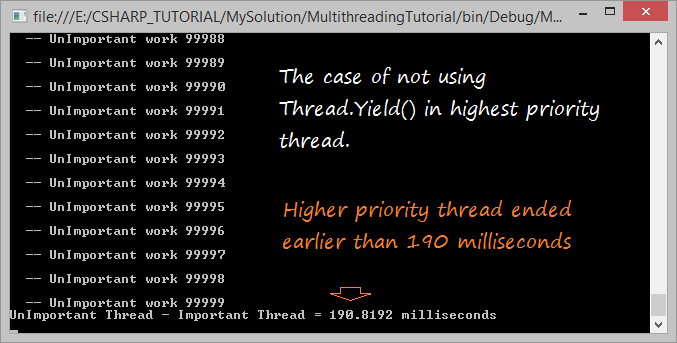Runs the above class in case the higher priority thread continuously calls Thread.Yield() to request that the system temporarily give priority to the other threads.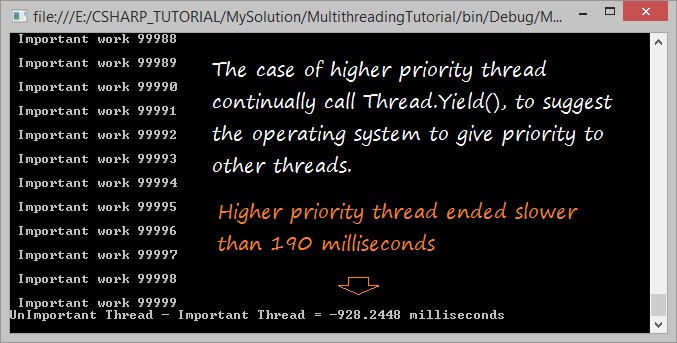#### View more Tutorials:

Maybe you are interested

These are online courses outside the o7planning website that we introduced, which may include free or discounted courses.